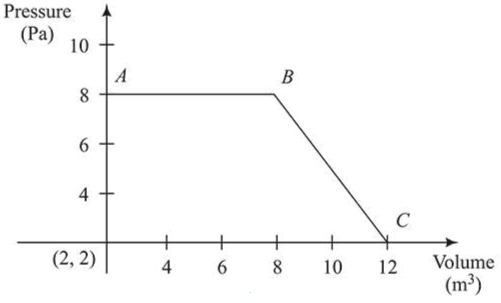# The magnitude of work done by a gas that undergoes a reversible expansion

Question:

The magnitude of work done by a gas that undergoes a reversible expansion along the path $A B C$ shown in the figure is ______________ .Solution:

(48.00)

Work done is given by the area under the trapezium.

$\therefore|\mathrm{w}|=\frac{1}{2}(6+10) \times 6=48 \mathrm{~J}$